# 1 Introduction

This vignette aims to demonstrate how to use the ‘ERP’ package for significance testing of event-related potentials (ERP) through the analysis of an example from a study investigating neural correlates of impulsive behavior (Shen, Lee, & Chen, 2014). ERPs are voltage changes along the scalp time-locked to some physical or mental events in the ongoing electrical brain activity recorded as electroencephalogram (EEG). ERPs are complex waveforms with highly correlated components in time corresponding to brief bursts of synchronized cortical activities. The current version of the ‘ERP’ package implements the adaptive factor-adjustment (AFA) procedure (Sheu, Perthame, Lee, & Causeur, 2016) and a generalized functional likelihood ratio test developed in Causeur, Sheu, Perthame, & Rufini (submitted) for detecting and identifying ERP signals. The procedure proposed by Guthrie & Buchwald (1991) for significance testing of difference potentials is also included in the package for comparison.

# 2 ERPs and impulsivity

Shen et al (2014) investigated the influence of impulsivity on response inhibition in a two-choice discrimination task using the stop-signal paradigm (Logan, Cowan, & Davis, 1984). In the study, participants were asked to withhold their responses when a ‘stop’ signal appeared after the ‘go’ stimulus. ERP amplitudes from 32 scalp locations (channels) were recorded from signal onset till 1,000 milliseconds (ms) after with one reading per 2 ms. For a stop trial, a participant either succeeded or failed to inhibit a response. Adult males who scored at the top 25% and bottom 25% on a scale measuring impulsive personality trait were recruited to participate in the experiment. There were twelve subjects in each impulsivity trait group (high or low).

The research questions to be addressed focus on the difference between the ERP curves from successful and failed response inhibition conditions: is this ‘condition’ effect significant? If so, is it the same at each scalp location? for the two trait groups? Is the difference between ERP curves located at the same time intervals in each channel and for each group?

For simplicity but with no loss of generality, in subsequent discussion we address the above questions by considering the scalp region consisting of only FCZ (fronto-central), CZ (central), and CPZ (centro-parietal) channels. Moreover, our demonstrations usually involves using the functions in the ‘ERP’ package in their default modes. The online help files give details for options not covered here.

# 3 Installing the package

The ERP package is available from the Comprehensive R Archive Network (CRAN) at https://cran.r-project.org/package=ERP. It can be installed by:

install.packages("ERP") 

The command below loads the data and functionality of the ERP package into the current R session:

library(ERP)

# 4 Importing impulsivity ERP data

The impulsivity data set comes with the ERP package as a data frame object of 144 rows by 505 columns.

data(impulsivity)
dim(impulsivity)
 144 505

ERP data sets arising from studies employing standard exprimental designs are typically stored in a specific format in which each row contains variables specifying information for a single ERP curve. For the ‘impulsivity’ data frame, they are listed as follows: channel index, subject index, treatment groups, experimental conditions (other covariates), and the amplititudes of ERP over sampling time points. The structure for the first six rows by eight columns of the ‘impulsivity’ data frame is shown below:

knitr::kable(head(impulsivity[, 1:8]))
Channel Subject Group Condition T_0 T_2 T_4 T_6
10 FCZ S11 High Success 0.084 -0.026 -0.111 -0.177
15 CZ S11 High Success 0.331 0.271 0.228 0.206
20 CPZ S11 High Success 0.712 0.722 0.741 0.774
40 FCZ S11 High Failure 0.689 0.609 0.521 0.425
45 CZ S11 High Failure -0.050 -0.160 -0.251 -0.318
50 CPZ S11 High Failure -0.306 -0.492 -0.656 -0.788

The ‘impulsivity’ data frame contains 144 (= 3 x 2 x 12 x 2) ERP curves from three channels, two response inhibition conditions (successful or failed), and 12 participants from each of the two impulsivity trait groups. The ERP amplitudes are recorded from 0 to 1,000 ms for every 2 ms and their values appear in 501 columns of the data object.

To facilitate subsequent steps in ERP data analysis, some frequently used values such as the number of curves (n), the time points sampled in milliseconds (time_pt) and the number of time frames (T) are assigned and saved as R objects. The levels of subject and channel variables (both are of factor types) are re-ordered along numerical sequence for the former and fronto-posterior axis for the latter.

time_pt <- seq(from = 0, to = 1000, by = 2)
T <- length(time_pt)
n <- nrow(impulsivity)
impulsivity$Subject <- factor(impulsivity$Subject,
levels(impulsivity$Subject)[c(1, 9, 20:24, 2:8, 10:19)]) impulsivity$Channel <- factor(impulsivity$Channel, levels(impulsivity$Channel)[c(3,2,1)])

The first 4 columns of the ‘impulsivity’ data frame contain experimental variables and the remaining 501 columns contain the amplitudes of ERPs. For convenience, we also save the design variables and the ERP curves, respectively, as ‘covariaes’ and ‘erpdata’ R objects.

covariates <- impulsivity[, 1:4]
erpdta <- impulsivity[, -(1:4)]  

The channel and condition are within-subject variables; whereas the trait group is a between-subject variable. For each combination of channel x condition x group, there are 12 ERP curves.

with(covariates, table(Channel, Condition, Group))
, , Group = High

Condition
Channel Failure Success
FCZ      12      12
CZ       12      12
CPZ      12      12

, , Group = Low

Condition
Channel Failure Success
FCZ      12      12
CZ       12      12
CPZ      12      12

# 5 Plotting ERP data and effect curves

## 5.1 Multipanel plots of ERP curves

First, we focus on the ‘condition’ effect of successful or failed response inhibition with respect to ‘stop’ signal trails as revealed by ERP curves. We start by graphing ERP curves with condition-specific colors in a plot of 3 (channel) by 2 (group) panels.

channels <- levels(covariates$Channel) groups <- levels(covariates$Group)
colors <- ifelse(covariates$Condition == "Success", "orange", "slateblue")  par(mfrow = c(3, 2)) for (i in 1:3) { for (j in 1:2) { select <- (covariates$Channel == channels[i]) &
(covariates$Group == groups[j]) erpplot(erpdta[select, ], frames = time_pt, col = colors[select], lty = 1, lwd = 2, cex.lab = 1.25, xlab = "Time (ms)", ylab = "ERP (mV)", main = paste("Channel: ", channels[i], " - Group: ", groups[j], sep = "")) } } legend("topright", bty = "n", lwd = 2, col = c("orange", "slateblue"), legend = c("Success", "Failure"))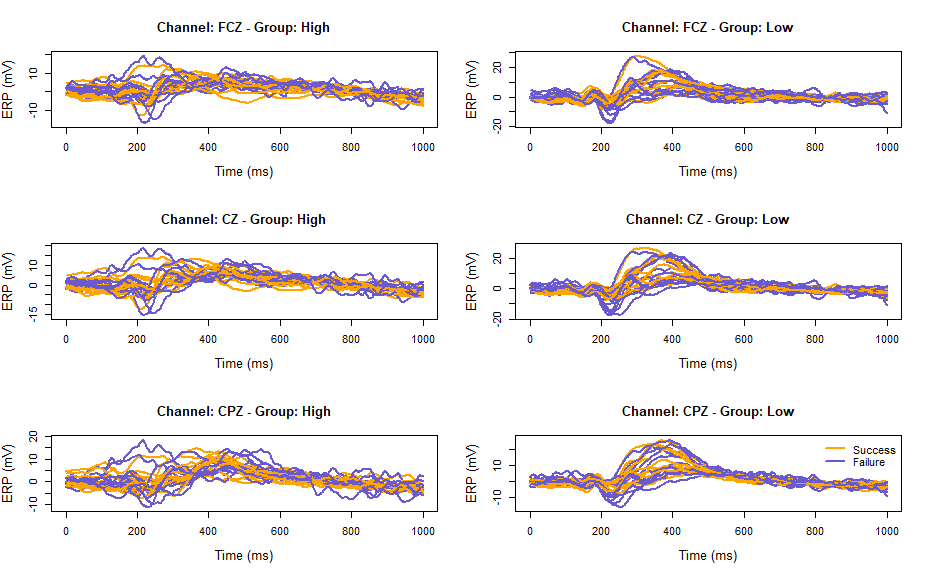Fig. 1: Successful (oragne) and failed (slate blue) inhibition ERP curves at FCZ, CZ and CPZ for high and low impulsivity groups. par(mfrow = c(1, 1)) ## 5.2 Plots of effect curves The analysis of ERP curves here aims primarily at testing for the ‘condition’ effect, i.e., difference between successful and failed response inhibition conditions, at each of three scalp locations for each of the two impulsivity groups, while accounting for the subject effect (individual difference). A linear model is formulated to account for these effects on the ERP curves as follow. Let $$Y_{ijkt}$$ be the amplitude of ERP curve observed at time $$t$$ in $$\left\{t_{1}, \ldots, t_{T=501}\right\}$$ (one recording for every 2 ms in the interval [0, 1000] ms after the stimulus onset) for subject $$i$$, $$i = 1, \ldots, 12$$, in condition $$j = 1, 2$$, group $$k = 1, 2$$ and at channel $$l = 1, 2, 3$$, then: $\begin{eqnarray*} Y_{ijklt} & = & \mu_{t} + \alpha_{it} + \beta_{jt} + \gamma_{kt} + \delta_{lt} + {\beta\gamma}_{jkt} + {\beta\delta}_{jlt} + {\gamma\delta}_{klt} + {\beta\gamma\delta}_{jklt} + \varepsilon_{ijkt} , \end{eqnarray*}$ where $$\alpha_{it}, \beta_{jt}, \gamma_{kt}$$ and $$\delta_{lt}$$ stand, respectively, for the main effect parameters of subject, condition, group and channel, the two-letter effect parameters for second-order interaction effects and the three-letter effect parameter for the third-order interaction effect at time $$t$$. The ‘condition’ effect is estimated by a difference curve between the mean ERPs in condition ‘Success’ and the mean ERPs in condition ‘Failure’. The model assumes that this effect curve may be different in the two impulsivity groups (condition $$\times$$ group interaction effect) and may also be different at the three channels (condition $$\times$$ channel interaction effect). It also assumes that the spatial distribution of the condition effect over the scalp may be different for the two impulsivity groups (condition $$\times$$ channel $$\times$$ group interaction effect). All these effects are to be tested. The design matrix of the linear model above can be obtained by the function ‘model.matrix’, using the standard symbolic expression for models in R: design <- model.matrix(~ C(Subject, sum)/Group + Group + Condition + Channel + Channel:Condition + Channel:Group + Condition:Group + Channel:Condition:Group, data = covariates) In order that all the effect parameters can be viewed as ‘mean’ effect curves over the subject, the ‘Subject’ effect parameters $$\alpha_{it}$$ are required to sum to 0 by the expression ‘C(Subject,sum)’. Because the two impulsivity groups have different subjects, the ‘Subject’ effect is embedded in the ‘Group’ effect, which is specified by ‘C(Subject,sum)/Group’. Note also that the default option for contrasts in R associated with a factor consists in setting the effect parameter of the first level (in alphanumeric order by default or in a specified factor order) to zero. Consequently, the condition effect curve $$t\mapsto\beta_{jt}$$ is constant for the reference level ‘Failure’ ($$j = 1$$) and it captures the ‘Success-Failure’ difference curve for $$j = 2$$, in group ‘High’ (the reference group) and at ‘FCZ’ (the reference channel after re-ording the channel levels from front to back). It can be checked that the ‘Success-Failure’ difference curve corresponds to the 26th column of the design matrix: colnames(design)[c(1, 23:27)]  "(Intercept)" "C(Subject, sum)22" "C(Subject, sum)23"  "GroupLow" "ConditionSuccess" "ChannelCZ"  We plot the ‘Success-Failure’ fitted difference curve using ‘erpplot’ with spline smoothing (the number of B-splines, ‘nsb’, has to be specified). Both pointwise (gray) and simultaneous (light gray) confidence intervals are shown: erpplot(erpdta, design, effect = 26, interval = "simultaneous", nbs = 20, lwd = 2, frames = time_pt, xlab = "Time (ms)", ylab = "Condition effect") title("Success-Failure difference curve \n Group High, Channel FCZ")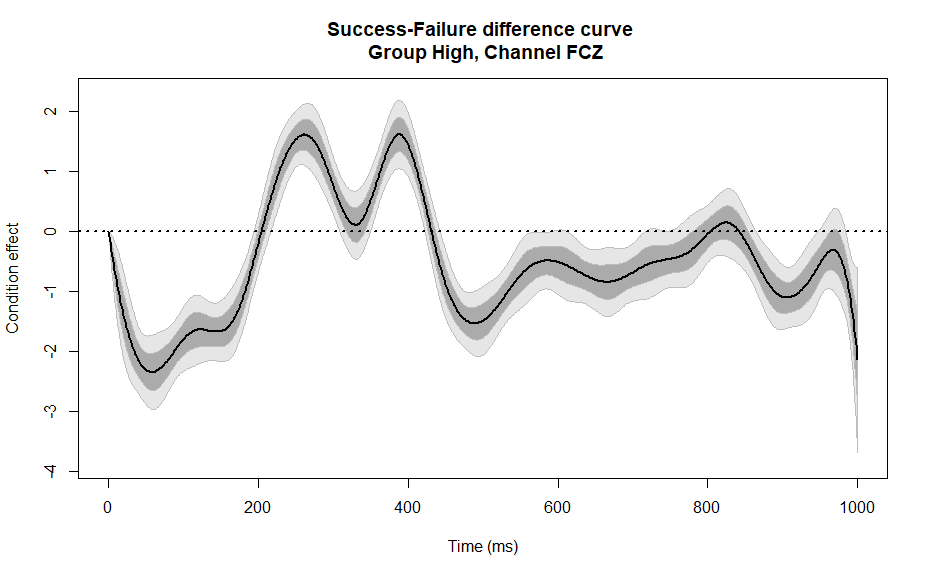Fig. 2: Difference ERP curve between response inhibition conditions at FCZ for high impulsivity group To examine all difference curves over channels and groups, the above R statements can be repeatedly applied after changing only the reference levels of the factors ‘Channel’ and ‘Group’: par(mfrow = c(3, 2)) for (i in 1:3) { for (j in 1:2) { covariates$Channel <- relevel(covariates$Channel,ref = channels[i]) covariates$Group <- relevel(covariates$Group,ref = groups[j]) design <- model.matrix(~ C(Subject, sum)/Group + Group + Condition + Channel + Channel:Condition + Channel:Group + Condition:Group + Channel:Condition:Group, data = covariates) erpplot(erpdta, design, effect = 26, interval = "simultaneous", nbs = 20, lwd = 2, frames = time_pt, ylim = c(-6, 6), xlab = "Time (ms)", ylab = "Condition effect") title(paste("Group ", groups[j], " Channel ", channels[i], sep = "")) } }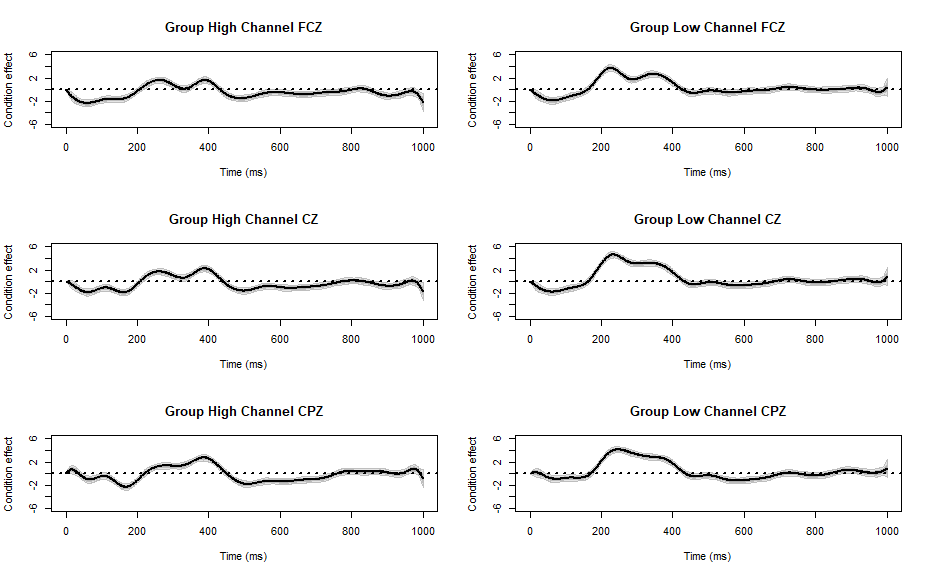Fig.3: Success-Failure difference curve by group for three channels par(mfrow = c(1, 1))  All the above ‘condition’ effects curves show a positive peak around 300 ms. Such peaks appear more pronounced in the ‘Low’ impulsivity group at all three locations. The first hypothesis to be tested in the following section is the impulsivity group comparison of the spatial variations of the ‘condition’ effect curves over the channels; i.e., the ‘Condition x Channel x Group’ interaction effect. # 6 Signal detection: significance of effect curves ERP amplitudes are measured in milliseconds for up to several seconds with reference to the onset of an event, the response of a subject to a stimulus (or a treatment) is a high resolution curve describing her differential cortical activity in time. As in traditional multivariate analysis in which the response provides a steady description of the subject through a small number of variables, functional data are similarly analyzed for covariate effects, both in experiment designs and in measuring effect sizes by p-values. For example, functional analysis of variance (fANOVA) extends standard analysis of variance to situations where the response is a curve (see Zhang, 2013 for a review). For significance testing of the mean difference of groups of curves, the one-way design is the most common. To cover a broad spectrum of experimental designs in ERP studies, the general framework of the present ERP package is a time-varying coefficient multivariate regression model with fixed-time covariates. ## 6.1 Functional Analysis of Variance of ERP curves Significance testing of an effect in a linear model framework is conducted via the F-test comparing the model including terms for the effect to be tested and the so-called null model obtained by setting the parameters specifying the effect to be tested to zero. To test for the ‘Condition x Channel x Group’ interaction effect, the design matrix of the null model is obtained as a submatrix of the design matrix ‘design’ by excluding the third order interation parameter: design0 <- model.matrix( ~ C(Subject, sum)/Group + Group + Condition + Channel + Channel:Condition + Channel:Group + Condition:Group, data = covariates) The function ‘erpFtest’ implements a functional F-test presented in Causeur, Sheu, Perthame, & Rufini (submitted), which accounts for the strong time dependence across the residuals of the model. The complexity of time dependence is handled by adjusting the number of factors in the factor model. The functional F-test assumes time independence when the number of factors is zero. Therefore, the first step of the testing procedure is to select the number of factors between 0 and the residual degrees of freedom of the model, in which case the regression factor model is saturated. Specifying ‘nbf = NULL’ and ‘pvalue = “none”’ for the function ‘erpFtest’ means that no calculation of p-values are expected in this step. The procedure introduced by Friguet, Kloareg, & Causeur (2009) to determine the number of factors for testing issues in regression factor model is implemented in the package. F <- erpFtest(erpdta, design, design0, nbf = NULL, pvalue = "none", wantplot = TRUE)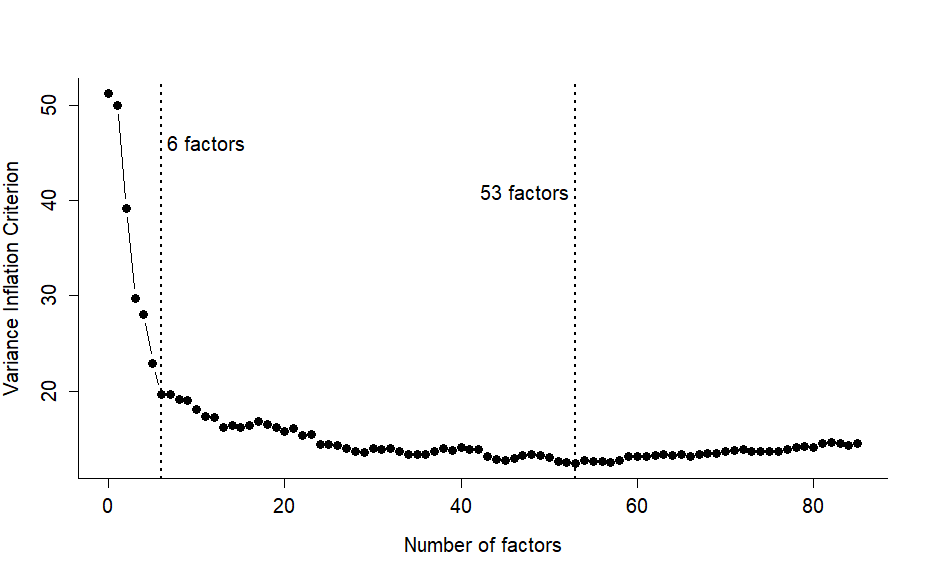Fig. 4: Variance Inflation Curve - Diagnostic plot to determine the number of factors in correlation-adjusted testing procedures. The variance inflation criterion (VIF) is plotted along the number of factors, reaching a minimum at which the corresponding number of factors is selected for the model. Regardless of the criterion chosen for determing the number of factors for a model given data, the shape of the curve on which the decision is based must be interpreted with great care. As in most scree plots, an obvious global minimum rarely occurs. The typical variance inflation curve decreases rapidly first, then more slowly and finally increases. A rigid adherenece to the decision rule consisting in choosing the value at which VIF is minimal may lead to retaining a very large number of factors for the model, thereby increasing the risk of overfitting; consequently, yielding too liberal a test, in which the type-I error level is left un-controlled. A rule of thumb that has been implemented in ‘erpFtest’ identifies the lowest number of factors $$q$$ for which the decrease in the criterion with respect to the preceding factor model with $$q-1$$ factors does not exceed 5% of the largest value of the criterion. The resulting value is provided in the ‘nbf’ component of the output of ‘erpFtest’. From the output given above, the recommended number of factors is: F$nbf
 6

We can go on to the next step of testing procedure with the number of factors selected. Alternaltively, to gain power, one might prefer inspecting the plot to choose a number between that from the output of ‘erpFtest’ and the value from the minimization of the criterion. Hereafter, we proceed with the smallest value for the number of factors recommended by the procedure. The function ‘erpFtest’ is again called with either ‘pvalue = “MC”’ or ‘pvalue = “TRUE”’, the default option. The latter option returns a p-value based on the Satterthwaite approximation of the null distribution by a $$c \chi^{2}_{\nu}$$ distribution; the former, a p-value obtained from a Monte-Carlo approximation of the null distribution. Although the latter supposedly provides a more accurate approximation of the null distribution than the Satterthwaite approximation, it requires at least 1,000 calculations of the functional F-test after random permutations of the rows of the design matrix (‘nsamples = 1000’), which can take a long time when the sample size and the number of time frames are large. Moreover, the Monte-Carlo method cannot estimate properly p-values lower than 1/nsamples. The p-value of the functional F-test is shown.

erpFtest(erpdta, design, design0, nbf = F$nbf)$pval 
 1

No significant third-order interaction effect is found. In other words, we can consider the spatial distribution of the condition effect to be the same for the two impulsivity groups.

## 6.2 Selecting significant effects in an ANOVA design

Each of the three second-order interaction effects can likewise be tested, starting from the design matrix in which all the interaction effects are present:

design <- model.matrix(~ C(Subject, sum)/Group + Group + Condition +
Channel + Channel:Condition + Channel:Group +
Condition:Group, data = covariates)

and deriving the design matrix of the null model by sequentially excluding one of the three interaction effects:

design0 <- model.matrix(~ C(Subject, sum)/Group + Group + Condition +
Channel + Channel:Group + Condition:Group,
data = covariates)
erpFtest(erpdta, design, design0, nbf = F$nbf)$pval  
 0.972

The ‘Condition x Channel’ interaction effect is tested first, followed by the ‘Group x Channel’ interaction effect, and then the ‘Condition x Group’ interaction effect.

design0 <- model.matrix(~ C(Subject, sum)/Group + Group + Condition +
Channel + Channel:Condition + Condition:Group,
data = covariates)
erpFtest(erpdta, design, design0, nbf = F$nbf)$pval  
 0.982
design0 <- model.matrix(~ C(Subject, sum)/Group + Group + Condition +
Channel + Channel:Condition + Channel:Group,
data = covariates)
erpFtest(erpdta, design, design0, nbf = F$nbf)$pval 
 9.26e-12

Note that we have kept the same number of factors used previously for testing the third-order interaction effect. Since the residual error of the model changes when the third-order interaction effect is removed, the number of factors should have been updated by setting the ‘nbf’ argument to ‘NULL’ in ‘erpFtest’. This also implies using different numbers of factors in each of the three tests above. In the present case, we have re-calculated the number of factors case by case and have gotten the same conclusions reported above.

From the results of the above functional F-tests only the ‘Condition x Group’ interaction effect is found to be significant, which means that, at each channel, the condition effect differs in the two impulsivity groups. This is consistent with the condition effect curves in panels of Fugure 3, in which the peak around 300 ms appears to be much more salient in the ‘Low’ impulsivity group.

Likewise, the difference between ERP curves at the three channels can be tested starting from the design matrix in which all the main effects and the ‘Condition x Group’ interaction effect are present:

design <- model.matrix(~ C(Subject, sum)/Group + Group + Condition +
Channel + Condition:Group, data = covariates)

and deriving the design matrix of the null model by removing the ‘Channel’ effect:

design0 <- model.matrix(~ C(Subject, sum)/Group + Group + Condition +
Condition:Group, data = covariates)
erpFtest(erpdta, design, design0, nbf = F$nbf)$pval  
 1.12e-09

Based on the p-value, the mean ERP curves are found to be significantly different in the three channels.

## 6.3 Fitted effect curves with the optimal model

The model corresponding to the last version of the design matrix, in which all the non-significant effects have been excluded, is used to explore more thoroughly the condition effect and to identify significant intervals. The effect curves, based on the model resulting from the functional ANOVA selection of the significant effects, is generated as follows:

par(mfrow = c(2,1))
for (i in 1:2) {
covariates$Group <- relevel(covariates$Group, ref = groups[i])
design <- model.matrix(~ C(Subject, sum)/Group + Group + Condition +
Channel + Condition:Group, data = covariates)
erpplot(erpdta, design, effect = 26, interval = "simultaneous",
lwd = 2, frames = time_pt, ylim = c(-6, 6),
xlab = "Time (ms)", ylab = "Condition effect")
title(paste("Success-Failure difference curve \n Group ",
groups[i], sep = ""))
}
par(mfrow = c(1, 1)) 

Note that, in the design matrix above, the contrast option for the channel effect is modified by introducing the expression ‘C(Channel, sum)’, which forces the sum of channel effect parameters to zero. Consequently, the other effect parameters can be viewed as mean curves over the channels.

Finally, the difference curves in the two groups are diplayed by cycling over the rows of the matrix ‘beta’ of fitted curves:

# 7 Signal identification: significant time intervals

The analysis of ERP curves using functional ANOVA in the previous section reveals a significantly different condition effect in the two impulsivity groups. One question remains: in which time intervals is the condition effect curve in a given group at a scalp location significant?

Here, we address this signal identification issue by examining ERPs at channel CPZ for subjects in high impulsivity group. First, we extract the corresponding ERP curves and covariates:

impulsivity.hcpz <- subset(impulsivity,
(impulsivity$Channel == "CPZ") & (impulsivity$Group == "High"))
impulsivity.hcpz <- droplevels(impulsivity.hcpz)
covariates.hcpz <- impulsivity.hcpz[, 1:4]
erpdta.hcpz <- impulsivity.hcpz[, -(1:4)]  

Comparing the ERP curves in the two response inhibition conditions at each time point is accomplished by pointwise t-tests in which the null model is obtained by setting the ‘Condition’ effect parameter to zero:

design <- model.matrix(~ C(Subject, sum) + Condition, data = covariates.hcpz)
design0 <- model.matrix(~ C(Subject, sum), data = covariates.hcpz)

Simultaneous tests at each time point are implemented to identify those time points for which the ‘Condition’ effect curves in the two impulsivity groups are significantly different from zero. The methods implemented in the ‘ERP’ package differ in how they control for the risk of an erroneous identification of a significant time point (false positive) and time dependence across pointwise tests. The components in the output are:

• $${\}$$signal contains the fitted effect curve(s)
• $${\}$$test contains the pointwise F-tests
• $${\}$$signif contains the indices of the significant time points

## 7.1 Averaging ERP curves in preselected time intervals

The function ‘erpavetest’ provides a basic method to address the simultaneous testing issue by partitioning the whole time frame into a preselected number of intervals with same length and averaging the ERPs of each single curve over the time points in each bin of the partition. The number of simultaneous tests is reduced from $$T$$ to the number of bins in the partition. The default option in ‘erpavetest’ introduces a partition of the whole time frame in ten intervals with equal length:

avetest <- erpavetest(erpdta.hcpz, design, design0)

The output ‘avetest’ is used to plot the ‘Condition’ effect curve:

erpplot(erpdta.hcpz, design, effect = ncol(design), lwd = 2,
interval = "simultaneous", frames = time_pt, ylim = c(-6, 6),
xlab = "Time (ms)", ylab = "Condition effect")
title("Success-Failure difference curve \n Group High, Channel CPZ")
points(time_pt[avetest$significant], rep(0, length(avetest$significant)),
pch = 20, col = "goldenrod")
abline(v = time_pt[avetest$breaks], lty = 2, col = "darkgray")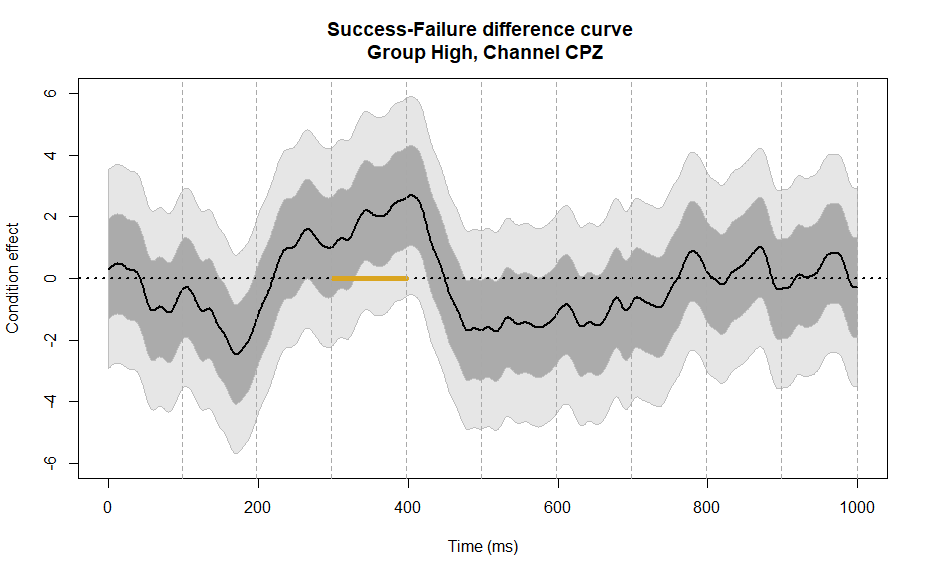Fig. 6: Fitted ‘Condition’ effect curve at channel CPZ for high impulsivity group with significant intervals identified by ‘erpavetest’. Dots corresponding to significant time points are colored in gold along the x-axis of the plot. A significant time interval [300, 400] ms is found. This procedure is however susceptible to ad hoc partitioning of time intervals. The alternative procedure presented below can be viewed as the extreme case of a partition in which each bin contains only one time point, leading to a set of poitwise test statistics over time. ## 7.2 Control of the False Discovery Rate The function ‘erptest’ implements pointwise F-tests with different options for controlling the risk of an erroneous identification of a significant time point. The default option is the Benjamini-Hochberg (BH) procedure (Benjamini and Hochberg, 1995), ensuring that the false discovery rate (FDR) is controlled at a preset level $$\alpha$$ (the default is $$\alpha=0.05$$). Other multiple testing procedures can be adopted by setting the argument ‘method’ to corresponding terms in ‘erptest’. bhtest <- erptest(erpdta.hcpz, design, design0) The output ‘bhtest’ is used to plot the ‘Condition’ effect curve: erpplot(erpdta.hcpz, design, effect = ncol(design), lwd = 2, interval = "simultaneous", frames = time_pt, ylim = c(-6, 6), xlab = "Time (ms)", ylab = "Condition effect") title("Success-Failure difference curve \n Group High, Channel CPZ") points(time_pt[bhtest$significant], rep(0, length(bhtest$significant)), pch = 20, col = "goldenrod")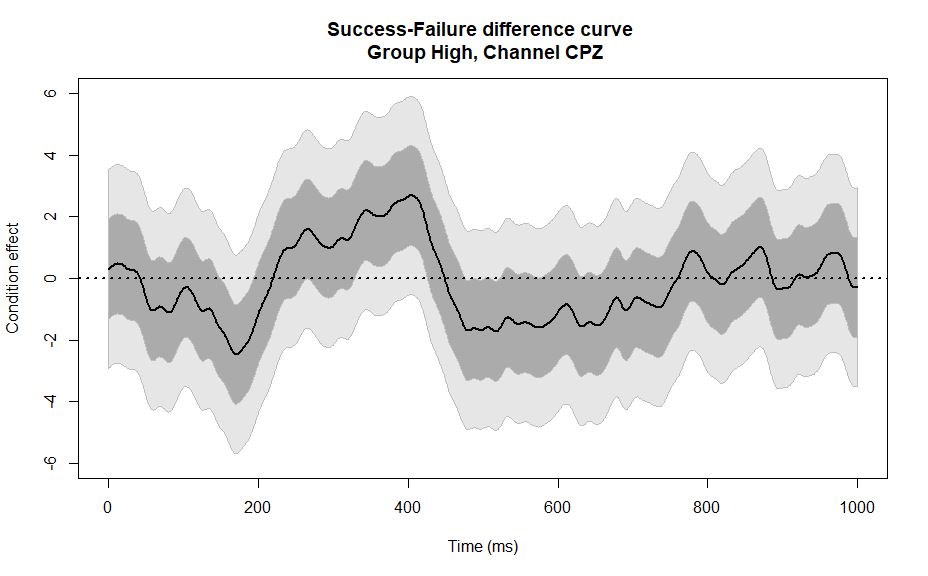Fig. 7: Fitted ‘Condition’ effect curve at channel CPZ for high impulsivity group with significant intervals identified by ‘erptest’. The BH procedure with a control of FDR at 0.05 level does not identify any significant time point. Sheu, Perthame, Lee & Causeur (2016) showed that the collection of pointwise test statistics inherit the strong temporal dependence among the residuals of the linear model. This dependence generates a pronounced regularity of this process of test statistics, which degrades both the power of the procedure and its accuracy, by generating spuriously low p-values outside of the support of the true effect curve. The next two methods explicitly accounts for this time dependence in the significance analysis. ## 7.3 The Guthrie-Buchwald procedure Although it is not explicitly designed to control the false discovery rate, the Guthrie-Buchwald procedure (Guthrie & Buchwald, 1991) partially accounts for time dependence by assuming a lag-1 autoregressive correlation model for the collection of pointwise test statistics. Indeed, once runs of successive significant time points are obtained using pointwise tests and a type-I error level $$\alpha = 0.05$$, only those sequences whose lengths are deemed too large with respect to the null distribution of significant run lengths of an autocorrelated process of test statistics are declared as significant time intervals. The function ‘gbtest’ implements this method with a default ‘graphical threshold’ of 0.05 (the graphical threshold is the type-I error level used to define a maximum run length over which an interval is declared significant). Note that the null distribution of run lengths is obtained using a Monte-Carlo method, which can require long calculation time for data sets with large designs. gb <- gbtest(erpdta.hcpz, design, design0) The output ‘gb’ is used to plot the ‘Condition’ effect curve: erpplot(erpdta.hcpz, design, effect = ncol(design), lwd = 2, interval = "simultaneous", frames = time_pt, ylim = c(-6, 6), xlab = "Time (ms)", ylab = "Condition effect") title("Success-Failure difference curve \n Group High, Channel CPZ") points(time_pt[gb$significant], rep(0, length(gb$significant)), pch = 20, col = "goldenrod")Fig. 8:Fitted ‘Condition’ effect curve at channel CPZ for high impulsivity group with significant intervals identified by ‘gbtest’. The Guthrie-Buchwald procedure fails to identify any significant intervals. This illustrates the conservatism of this method. ## 7.4 The Adapative Factor-Adjustment method Sheu et al. (2016) observed that the time dependence structure in ERP data is more complex than a lag-1 autoregressive correlation structure, with intervals of more strongly inter-dependent time points. This motivates their proposal for a more flexible adapative factor-Aadjustment (AFA) multiple testing procedure to disentangle the expected process of test statistics from the time dependent noise. As with the functional ANOVA testing procedure, the choice of the number of factors is handled by the same minimization of the variance inflation criterion, using the function ‘erpfatest’ with argument ‘nbf = NULL’: fabh <- erpfatest(erpdta.hcpz, design, design0, nbf = NULL, wantplot = TRUE)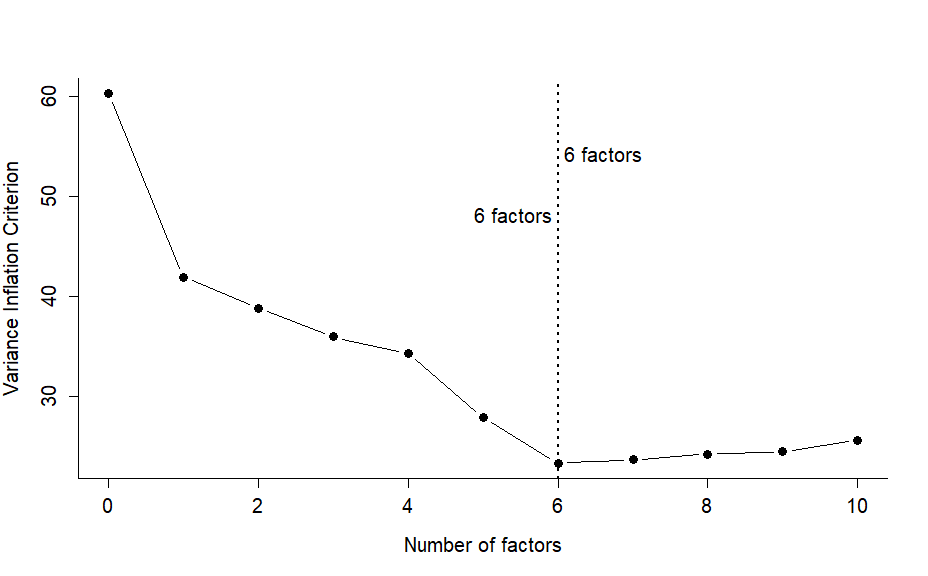Fig. 10: Variance Inflation diagnostic plot to choose the number of factors in the AFA method nbf <- fabh$nbf

The shape of the variance inflation vriterion curve is rather similar to the one observed for the functional ANOVA approach of the previous section. We also choose to proceed with the multiple testing procedure using the smallest recommended number of factors.

The function ‘erpfatest’ is again called with the chosen number of factors. The defaults for the multiple testing procedure are the same as those for the function ‘erptest’ (BH with a control of FDR at level 0.05).

fabh <- erpfatest(erpdta.hcpz, design, design0, nbf = nbf)

For the series of pointwise test statistics, the least-squares estimation of the effect curve may also be affected by a too strong regularity due to the strong time dependence among the residuals of the linear model. The component ‘fattest$signal’ contains a correlation-adjusted estimation of the effect curve developed in Causeur, Sheu, Perthame & Rufini (submitted): erpplot(erpdta.hcpz, design, effect = ncol(design), lwd = 2, interval = "simultaneous", frames = time_pt, ylim = c(-6, 6), xlab = "Time (ms)", ylab = "Condition effect curve") title("Success-Failure difference curve \n Group High, Channel CPZ") points(time_pt[fabh$significant], rep(0, length(fabh\$significant)),
pch = 20, col = "goldenrod")